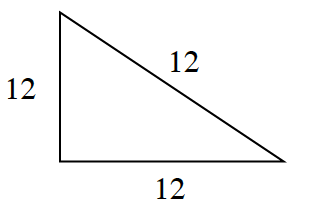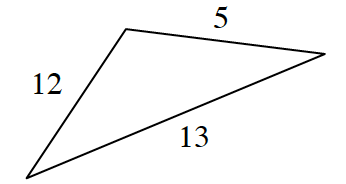### Home > GC > Chapter 12 > Lesson 12.2.1 > Problem12-58

12-58.

Examine the triangles below. Decide if each one is a right triangle. If the triangle is a right triangle, justify your conclusion. Assume that the diagrams are not drawn to scale.1.What are the angle measures of an equilateral triangle?

No, all the angles must be $60^\circ$.

1.Use the Pythagorean Theorem to check. Remember, the hypotenuse is the longest side.

Yes, the side lengths are a Pythagorean Triple.